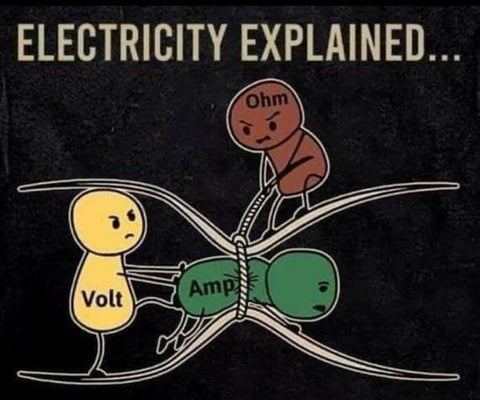# Why does voltage matter so much on my golf cart?

Written by Gregory PeresOften the basic statement is given:
"Ampere (amps) (A) = torque. Voltage (V) = speed."
Its actually not totally correct statement per-say and to be exact:
"Wattage = torque" is a more accurate way to say it.
It all boils down to Ohms law: Current (I) = Voltage (V) / Resistance (R)
Say we have a common garden hose. We want more water to come out of it we can increase the pressure (voltage) and more water will flow (current). Increase the diameter of the hose and more water will come out of the hose (resistance).
Power is measured in watts. In terms of electricity Power (P) = Voltage (V) * Current (I)
So take the common hose and point it at a water wheel you can increase the power the wheel generates in two different ways.
1. Increase the pressure (voltage) of the water coming out of the hose and hit it with more force you will increase the power the waterwheel generates.
2. Increase the flow of water (current) by using a bigger hose (decrease resistance) and hit the waterwheel with more weight from the water it will increase the power it generates.
In an electrical system increasing either the current or the voltage will result in higher power too! This is why going from 36v to 48v to 72v makes your cart faster and use less current to do it!
Demonstrated by the equation: Current (I) = Power (P) / Voltage (V)
So lets take the 5000w (5kW) Navitas AC Inductive motor as a base for Power (P).
A 36v lead acid pack when fully charged at 100% SoC is roughly 38.22V. 48v pack is 50.96V. 72v pack is 76.44V.
At 38.22V it takes 130.82A of current to satisfy 5000w of Power (P)
At 50.96V it takes 98.12A of current to satisfy 5000w of Power (P)
At 76.44V it takes 65.41A of current to satisfy 5000w of Power (P)
What you say? It takes less current to get to 5000w at a higher voltage! Yes! In fact... a 72v pack takes half the Current (I) of a 36v pack to do the same thing!
Doing the math: 130.82A / 65.41A = 2
To be explicit it takes half the current to generate the same 5000w of power @ 72v! The efficiency of electric motors improves at higher voltages!!
This is why the EV after market is consistently increasing the voltage of their setups. This is also why the golf cart / LSV market is moving towards 72v as a standard as well.
So before paying big bucks on your pricy lithium setup seriously consider 72v over 48v. It takes less current @ 72v to do the same thing at 48v.